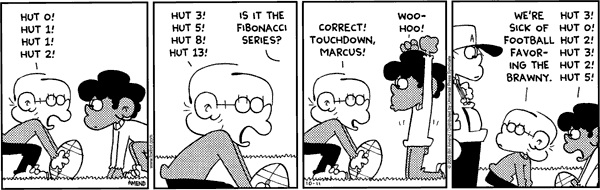## On To The Next…

Another year almost over, the next one about to begin. Which makes me think of sequences…

Math jokes make all my Cauchy sequences converge.

And here’s a Fox Trot cartoon with sequences:You undoubtedly can identify the first sequence: 1, 1, 2, 3, 5, 8, 13, …
Of course, it’s the Fibonacci sequence.

But do you recognize the sequence from the last panel? It begins 3, 0, 2, 3, 2, 5, …

If not, here’s your first question:

What’s the next term in that sequence?

What is the general formula for the terms in that sequence? (A recursive formula is completely acceptable. The explicit formula is quite a beast.)

Like the Fibonacci sequence, this sequence is defined by a recurrence relation. In particular,

P(0) = 3, P(1) = 0, P(2) = 2, and P(n) = P(n – 2) + P(n – 3)

This sequence has an amazing property: For any natural number n, if n is prime, then n | P(n). No, really. You can check for yourself. P(3), P(5), and P(7) are trivial, since P(3) = 3, P(5) = 5, and P(7) = 7. But…

P(11) = 22, and 11|22

P(13) = 39, and 13|39

P(17) = 119, and 17|119

P(19) = 209, and 19|209

P(23) = 644, and 23|644

Also like the Fibonacci sequence, the ratio of consecutive numbers in this sequence have a constant ratio. As we all know, the ratio of consecutive Fibonacci numbers is approximately 1.618034, better known as the golden ratio. For the Perrin sequence, the ratio of consecutive numbers is approximately 1.324718, known as the plastic constant.

Cool stuff.

Here are a couple other sequences for you to ponder as you prepare for the new year. Can you determine the next term?

O, T, T, F, F, S, S, E, …

3, 3, 5, 4, 4, 3, 5, 5, …

6, 14, 24, 36, 50, &hellip;

Entry filed under: Uncategorized. Tags: , , , .

• 1.xander  |  December 30, 2010 at 1:58 am

O, T, T, F, F, S, S, E: The next terms are N, T, E. These are the initials of the counting numbers. (O)ne, (T)wo, (T)hree, and so on.

3, 3, 5, 4, 4, 3, 5, 5: The next terms are 4, 3, 6. S(n) = the number of letters in the word for the number n. One has three letters, two has three letters, &c.

6, 14, 24, 36, 50: I think that the next terms are 66, 94, 114. a_0 = 0, a_{n+1} = a_{n} + 2(n+3). I think. Maybe. It has been a while since my theory of positive integers, so I don’t think that I am going to try to work out an explicit formula right now, though I don’t think that it should be that difficult. Call me lazy. 😉